Select Page

# Application of Integrals Maths 12 Science CBSE Answers for MCQ in English

Application of Integrals Maths 12 Science CBSE Answers for MCQ in English to enable students to get Answers in a narrative video format for the specific question.

Expert Teacher provides Application of Integrals Maths 12 Science CBSE Answers for MCQ through Video Answers in English language. This video solution will be useful for students to understand how to write an answer in exam in order to score more marks. This teacher uses a narrative style for a question from Application of Integrals not only to explain the proper method of answering question, but deriving right answer too.

Please find the question below and view the Answer in a narrative video format.

Question:

Answer Video in English:

## Similar Questions from CBSE, 12th Science, Maths, Application of Integrals

Question 1 : Find the area of the region bounded by the parabolaand y = | x |. (View Answer Video)

Question 2 : Find the area enclosed by the parabolaand the line x - y = 4. (View Answer Video)

Question 3 : Find the area of smaller region bounded by the ellipseand the line. (View Answer Video)

Question 4 : Given that, for x = 0 to x = 20. Find f(x) and g(x) such that the area between f(x) andfrom x = 0 to x = 5 beand area between g(x) andfrom y = 0 to y = 5 be. Is? Like functions f and g which work is better, team work or individual work? (View Answer Video)

Question 5 : Using the method of integration find the area bounded by the curve |x| + |y| = 1. (View Answer Video)

### Differential Equations

Question 1 : Find the particular solution of the differential equationgiven that y = 0, when x = 0. (View Answer Video)

Question 2 : Determine degree of(View Answer Video)

Question 3 : Find the general solution of differential equation(View Answer Video)

Question 4 : Write the degree of the differential equation(View Answer Video)

Question 5 : Find the particular solution of the differential equationgiven that y = 1 when x = 0. (View Answer Video)

### Inverse Trigonometric Functions

Question 1 : Write the principal value of(View Answer Video)

Question 2 : Evaluate :(View Answer Video)

Question 3 : Ifthen value of y lies between.....? (View Answer Video)

Question 4 : Solve for(View Answer Video)

Question 5 : Evaluate :(View Answer Video)

### Probability

Question 1 : If P(A)=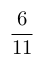, P(B)=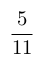and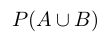=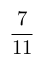, find. (View Answer Video)

Question 2 :  If E and F are independent events, then show that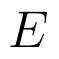and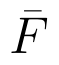Question 4 : If P(A)=0.8, P(B)=0.5 and=0.4, find. (View Answer Video)
Question 5 :  A die is rolled. If E= (1, 3, 5), F(2, 3) and G= {2, 3, 4, 5}, find(View Answer Video)